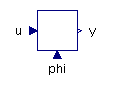Two Way Valve - MapleSim Help

Two-Way Two-Position Valve

Two-position valve that controls flow between two portsDescription

The Two-Way Two-Position Valve component allows flow between ports A and P with two positions to control the flow.

The real input $s$ controls the position of the value. An input of 0 corresponds to position 0, an input of 1 to position 1. Intermediates values produce an averaged position, with the transition length set by the $\mathrm{band}$ parameter.

The following figure shows how $s$ and $\mathrm{band}$ effect the valve opening.Formulation Approaches Two approaches were taken for formulation of the flow equation inside the device. When the boolean parameter $\mathrm{Use constant Cd}$ is true, a constant coefficient of discharge (${C}_{d}$) is used, otherwise a variable coefficient of discharge with maximum value (${C}_{d\left(\mathrm{max}\right)}$) and a critical flow number (${\mathrm{Crit}}_{\mathrm{no}}$) are used.Connections

 Name Description Modelica ID $\mathrm{portA}$ Hydraulic port to path A portA $\mathrm{portP}$ Hydraulic port for pump portP $s$ Real input signal that controls valve position sParametersGeneral

 Name Default Units Description Modelica ID ${A}_{\mathrm{max}}$ $1·{10}^{-4}$ ${m}^{2}$ Maximum orifice opening area Amax ${A}_{\mathrm{min}}$ $1·{10}^{-12}$ ${m}^{2}$ Minimum orifice opening area (leakage) Amin $\mathrm{band}$ $0.1$ Transition length in normalized spool displacement bandOrifice

 Name Default Units Description Modelica ID $\mathrm{Use constant Cd}$ $\mathrm{true}$ True (checked) means a constant coefficient of discharge is implemented, otherwise a variable ${C}_{d}$ is used in flow calculation UseConstantCd ${C}_{d}$ $0.7$ Flow-discharge coefficient; used when $\mathrm{Use constant Cd}$ is true Cd ${\mathrm{\Re }}_{\mathrm{Cr}}$ $12$ Reynolds number at critical flow; used when $\mathrm{Use constant Cd}$ is true ReCr ${C}_{d\left(\mathrm{max}\right)}$ $0.7$ Maximum flow-discharge coefficient; used when $\mathrm{Use constant Cd}$ is false Cd_max ${\mathrm{Crit}}_{\mathrm{no}}$ $1000$ Critical flow number; used when $\mathrm{Use constant Cd}$ is false Crit_noOptional Volumes

 Name Default Units Description Modelica ID $\mathrm{Use volume A}$ $\mathrm{false}$ True (checked) means a hydraulic volume chamber is added to portA useVolumeA ${V}_{A}$ $1·{10}^{-6}$ ${m}^{3}$ Volume of chamber A Va $\mathrm{Use volume P}$ $\mathrm{false}$ True (checked) means a hydraulic volume chamber is added to portP useVolumeP ${V}_{P}$ $1·{10}^{-6}$ ${m}^{3}$ Volume of chamber P Vb